# PSAT Math : How to factor an equation

## Example Questions

2 Next →

### Example Question #11 : Equations / Inequalities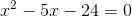Solve for.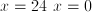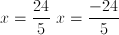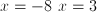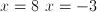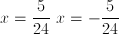Explanation:Find all factors of 24

1, 2, 3,4, 6, 8, 12, 24

Now find two factors that add up to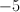and multiply to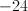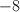and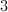are the two factors.

By factoring, you can set the equation to be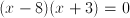If you FOIL it out, it gives you.

Set each part of the equation equal to 0, and solve for.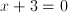and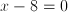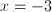and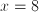### Example Question #11 : How To Factor An Equation

Assume thatandare integers and that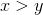. The value of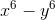must be divisible by all of the following EXCEPT: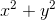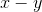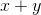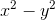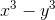Explanation:

The numbers by which x6 – yis divisible will be all of its factors. In other words, we need to find all of the factors of x6 – y6 , which essentially means we must factor x6 – yas much as we can.

First, we will want to apply the difference of squares rule, which states that, in general, a2 – b2 = (a – b)(a + b). Notice that a and b are the square roots of the values of a2 and b2, because √a2 = a, and √b2 = b (assuming a and b are positive). In other words, we can apply the difference of squares formula to x6 – y6 if we simply find the square roots of x6 and y6.

Remember that taking the square root of a quantity is the same as raising it to the one-half power. Remember also that, in general, (ab)c = abc.

x6 = (x6)(1/2) = x(6(1/2)) = x3

Similarly, √y6 = y3.

Let's now apply the difference of squares factoring rule.

x6 – y= (x3 – y3)(x3 + y3)

Because we can express x6 – y6 as the product of (x3 – y3) and (x3 + y3), both (x3 – y3) and (x3 + y3) are factors of x6 – y. Thus, we can eliminate x3 – yfrom the answer choices.

Let's continue to factor (x3 – y3)(x3 + y3). We must now apply the sum of cubes and differences of cubes formulas, which are given below:

In general, a3 + b3 = (b)(a2 – ab + b2). Also, a3 – b3 = (a – b)(a2 + ab + b2)

Thus, we have the following:

(x3 – y3)(x3 + y3) = (x – y)(x2 + xyy2)(x + y)(x2 – xy + y2)

This means that x – y and x + y are both factors of x6 – y, so we can eliminate both of those answer choices.

We can rearrange the factorization (x – y)(x2 + xyy2)(x + y)(x2 – xy + y2) as follows:

(x – y)(y)(x2 + xyy2)(x2 – xy + y2)

Notice that (x – y)(y) is merely the factorization of difference of squares. Therefore, (x – y)(y) = x– y2.

(x – y)(y)(x2 + xy +y2)(x2 – xy + y2) = (x2 – y2)(x2 + xy +y2)(x2 – xy + y2)

This means that x2 – y2 is also a factor of x6 – y6.

By process of elimination, x2 + y2 is not necessarily a factor of  x6 – y.

The answer is  x2 + y2 .

### Example Question #91 : Algebra

Factor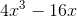.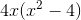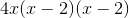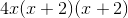Cannot be factored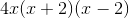Explanation:

First pull out any common terms: 4x3 – 16x = 4x(x2 – 4)

x2 – 4 is a difference of squares, so we can also factor that further. The difference of squares formula is a2 – b2 = (a – b)(a + b). Here a = x and b = 2. So x2 – 4 = (x – 2)(x + 2).

Putting everything together, 4x3 – 16x = 4x(+ 2)(– 2).

### Example Question #751 : Psat Mathematics

Factor the following: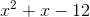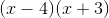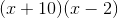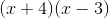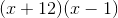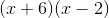Explanation:

Start by looking at your last term. Since this term is negative, you will need to have a positive group and a negative group: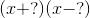Now, since the middle term is positive, you can guess that the positive group will contain the larger number. Likewise, since the coefficient is only, you can guess that the factors will be close.  Two such factors ofareand.### Example Question #11 : How To Factor An Equation

Factor the following: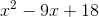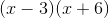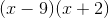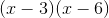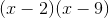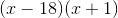Explanation:

Begin by looking at the last element. Since it is positive, you know that your groups will contain either two additions or two subtractions. Since the middle term is negative (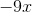), your groups will be two subtractions: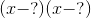Now, the factors ofareandand, andand.

Clearly, the last is the one that works, for when you FOIL, you get your original equation!

### Example Question #1981 : Sat Mathematics

Factor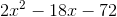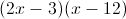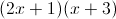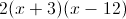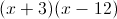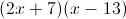Explanation:

We can factor out a, leaving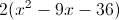.

From there we can factor again to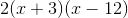.

2 Next →

### All PSAT Math Resources